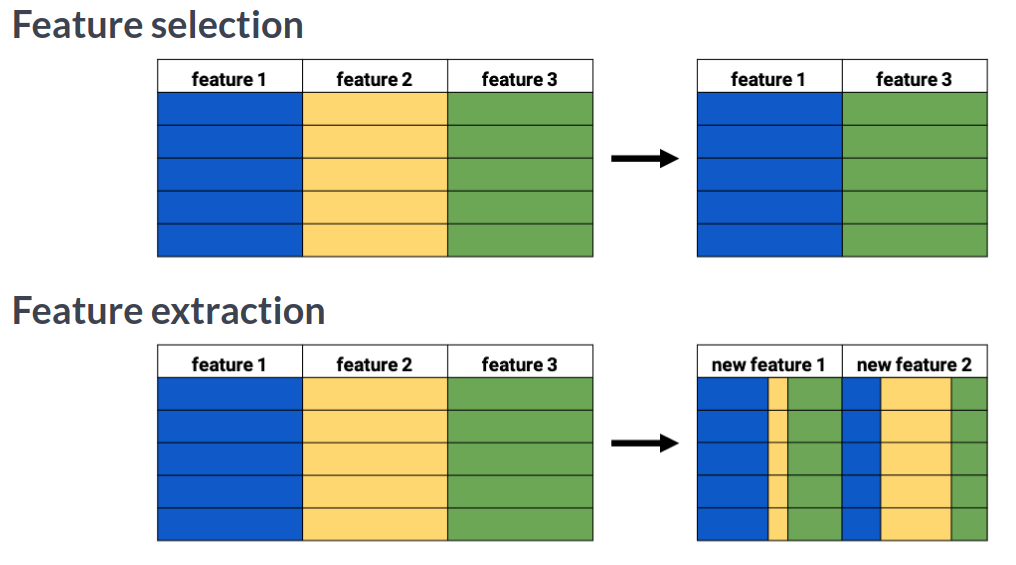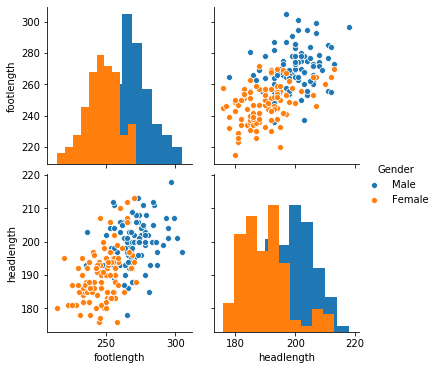import pandas as pd
import numpy as np
import matplotlib.pyplot as plt
import seaborn as sns

plt.rcParams['figure.figsize'] = (10, 5)


## Introduction

### Removing features without variance

A sample of the Pokemon dataset has been loaded as pokemon_df. To get an idea of which features have little variance you should use the IPython Shell to calculate summary statistics on this sample. Then adjust the code to create a smaller, easier to understand, dataset.

pokemon_df = pd.read_csv('./dataset/pokemon_gen1.csv')

HP Attack Defense Generation Name Type Legendary
0 45 49 49 1 Bulbasaur Grass False
1 60 62 63 1 Ivysaur Grass False
2 80 82 83 1 Venusaur Grass False
3 80 100 123 1 VenusaurMega Venusaur Grass False
4 39 52 43 1 Charmander Fire False
pokemon_df.describe()

HP Attack Defense Generation
count 160.00000 160.00000 160.000000 160.0
mean 64.61250 74.98125 70.175000 1.0
std 27.92127 29.18009 28.883533 0.0
min 10.00000 5.00000 5.000000 1.0
25% 45.00000 52.00000 50.000000 1.0
50% 60.00000 71.00000 65.000000 1.0
75% 80.00000 95.00000 85.000000 1.0
max 250.00000 155.00000 180.000000 1.0
number_cols = ['HP', 'Attack', 'Defense']

# Leave this list as is for now
non_number_cols = ['Name', 'Type', 'Legendary']

# Sub-select by combining the lists with chosen features
df_selected = pokemon_df[number_cols + non_number_cols]

# Prints the first 5 lines of the new dataframe

   HP  Attack  Defense                   Name   Type  Legendary
0  45      49       49              Bulbasaur  Grass      False
1  60      62       63                Ivysaur  Grass      False
2  80      82       83               Venusaur  Grass      False
3  80     100      123  VenusaurMega Venusaur  Grass      False
4  39      52       43             Charmander   Fire      False

number_cols = ['HP', 'Attack', 'Defense']

# Remove the feature without variance from this list
non_number_cols = ['Name', 'Type', ]

# Create a new dataframe by subselecting the chosen features
df_selected = pokemon_df[number_cols + non_number_cols]

# Prints the first 5 lines of the new dataframe

   HP  Attack  Defense                   Name   Type
0  45      49       49              Bulbasaur  Grass
1  60      62       63                Ivysaur  Grass
2  80      82       83               Venusaur  Grass
3  80     100      123  VenusaurMega Venusaur  Grass
4  39      52       43             Charmander   Fire


## Feature selection vs feature extraction

• Why reduce dimensionality?
• be less complex
• require less disk space
• require less computation time
• have lower chance of model overfitting### Visually detecting redundant features

Data visualization is a crucial step in any data exploration. Let's use Seaborn to explore some samples of the US Army ANSUR body measurement dataset.

ansur_df_1 = pd.read_csv('./dataset/ansur_df_1.csv')

sns.pairplot(ansur_df_1, hue='Gender', diag_kind='hist');reduced_df = ansur_df_1.drop('body_height', axis=1)

# Creat a pairplot and color the points using the 'Gender' feature
sns.pairplot(reduced_df, hue='Gender');sns.pairplot(ansur_df_2, hue='Gender', diag_kind='hist');reduced_df = ansur_df_2.drop(['n_legs'], axis=1)

# Create a pairplot and color the points using the 'Gender' feature
sns.pairplot(reduced_df, hue='Gender', diag_kind='hist');the body height (inches) and stature (meters) hold the same information in a different unit + all the individuals in the second sample have two legs.

## t-SNE visualization of high-dimensional data

### Fitting t-SNE to the ANSUR data

t-SNE is a great technique for visual exploration of high dimensional datasets. In this exercise, you'll apply it to the ANSUR dataset. You'll remove non-numeric columns from the pre-loaded dataset df and fit TSNE to his numeric dataset.

ansur_male = pd.read_csv('./dataset/ANSUR_II_MALE.csv')

df = pd.concat([ansur_male, ansur_female])

from sklearn.manifold import TSNE

# Non-numeric columns in the dataset
non_numeric = ['Branch', 'Gender', 'Component', 'BMI_class', 'Height_class']

# Drop the non-numeric columns from df
df_numeric = df.drop(non_numeric, axis=1)

# Create a t-SNE model with learning rate 50
m = TSNE(learning_rate=50)

# fit and transform the t-SNE model on the numeric dataset
tsne_features = m.fit_transform(df_numeric)
print(tsne_features.shape)

(6068, 2)


t-SNE reduced the more than 90 features in the dataset to just 2 which you can now plot.

### t-SNE visualisation of dimensionality

Time to look at the results of your hard work. In this exercise, you will visualize the output of t-SNE dimensionality reduction on the combined male and female Ansur dataset. You'll create 3 scatterplots of the 2 t-SNE features ('x' and 'y') which were added to the dataset df. In each scatterplot you'll color the points according to a different categorical variable.

df['x'] = tsne_features[:, 0]
df['y'] = tsne_features[:, 1]

sns.scatterplot(x='x', y='y', hue='Component', data=df);sns.scatterplot(x='x', y='y', hue='Branch', data=df);sns.scatterplot(x='x', y='y', hue='Gender', data=df);